# Antenna Directivity

Antenna Directivity is measured in correspondence to the concentration of the radiated energy by the transmitting antenna specifically in a particular direction. While for the receiving antenna, directivity represents the ability of the antenna to gather most of the radiated energy from a specific direction.

### Introduction

Directivity is associated with antenna gain and antenna efficiency. In other words, basically, antenna gain has two components, one is directivity while the other is efficiency.

Thus, the relationship is given as:

Antenna Gain = Antenna Directivity * Antenna Efficiency

This implies that the gain of the antenna is the product of directivity and efficiency of the antenna. Directivity is different for different types of antennas. Generally, for short dipole, its value is 1.76 dB while for large dish antenna it is around 50 dB.

It is also called maximum directive gain and is denoted by D.

## What is Directivity?

• Let us see the first way to define directivity.

Antenna Directivity is defined as the ratio of maximal radiation intensity to the average radiation intensity and both are considered for the test antenna.

Thus, it can be written as: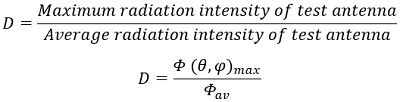Here, Φ(θ,φ)max represents the power per unit solid angle, denoting the maximum radiation intensity. And φav shows the average radiation intensity which is averaged over a spherical system.

Hence,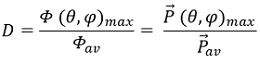So, in terms of power density, directivity is defined as the ratio of maximum power density to the average power density over a sphere where both are measured in the far-field of the test antenna.

Directivity is a dimensionless quantity whose value is generally equal to or greater than 1.

• Now, let us further see how the directivity is defined in another way.

Here the directivity is defined relative to the overall radiated power. Antenna directivity is alternatively defined as the ratio of overall power which is radiated by the subject antenna to the power radiated by the isotropic antenna where the radiation intensity is the same.

Hence, we can write it as: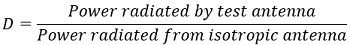So, when the intensity of emitted radiation is the same then,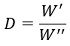Here, WI represents the power radiated by the test antenna and WII denotes the power radiated by the isotropic (omnidirectional) antenna.

As we know, that to get the average radiation intensity, total radiated power must be divided by 4π steradian.

Thus,This means the directivity is associated with maximal values of the antenna.Directivity is a crucial factor associated with antenna due to the reason that for transmitting antennas some systems specifically radiate in a particular direction by forming narrow-angle. While for receiving antenna, the directivity is checked as its ability to gather the radiated energy from a specific direction. This shows that it is concerned with the level of directivity possessed by the radiation pattern of the antenna.

• Hence is considered as the fundamental parameter of the antenna. But despite being the fundamental property of antenna all antennas do not possess high directivity still find their uses in various fields.

Generally, based on the directivity that an antenna exhibit, antennas are classified as low directivity and high directivity antenna.

An antenna which radiates in almost all the directions, where the only variation exists in the intensity of the radiation in the various directions has directivity of 0 or 1 dB. These antennas are known as an omnidirectional antenna. But in case of this antenna, the radiated beam propagates to shorter distances.

Basically, when an antenna possesses high directivity then the radiated beam is of extremely focused nature and thus propagates to longer distances in comparison to the radiated beam from low directivity antenna.

Now, as we have discussed in this same section that an antenna which possesses low directivity also finds major applications. This is so because high antenna directivity is not suitable in all applications. So, the first question arises then where it is used?

• Generally, antennas possessing high directivity are used in such applications where antennas are permanently installed. So, due to the fixed position, the radiated energy is required to be propagated to longer distance and this is facilitated by an antenna with high directivity. The main example of this type of antenna is a satellite TV antenna.
• But when we talk about low directivity antennas then its uses are associated with the circumstances where the antennas are not required to be installed at a specific place and can be mobile. This is so because in this case the mobile transmitting antennas can transmit the energy in all the specific directions and the mobile receiving antenna can gather the information from any direction coming towards it. Its example is the antenna for cell phones.

### Directive Gain and Directivity

We know that,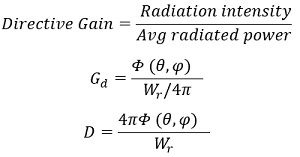The crucial difference between directive gain and directivity is that directive gain implies the extent up to which the antenna under test concentrates its radiated energy with respect to the standard antenna. While the maximum directive gain of the antenna is defined as directivity.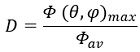Another expression is,When the lossless isotropic antenna is assumed then, the directive gain is equal to directivity.

So,: k is the radiation efficiency factor whose value is 1 for the isotropic antenna.